# Algebraic Division

## Introduction

The process for dividing one polynomial by another is very similar to that for dividing one number by another.

There are two ways to divide polynomials but we are going to concentrate on the most common method here: The algebraic long method or simply the traditional method of dividing algebraic expression.

## Algebraic Long Method

Here are the steps in dividing polynomials using the long method:

1. Arrange the indices of the polynomial in descending order. Replace the missing term(s) with 0.
2. Divide the first term of the dividend (the polynomial to be divided) by the first term of the divisor. This gives the first term of the quotient.
3. Multiply the divisor by the first term of the quotient.
4. Subtract the product from the dividend then bring down the next term. The difference and the next term will be the new dividend. Note: Remember the rule in subtraction "change the sign of the subtrahend then proceed to addition".
5. Repeat step 2 – 4 to find the second term of the quotient.
6. Continue the process until a remainder is obtained. This can be zero or is of lower index than the divisor.

If the divisor is a factor of the dividend, you will obtain a remainder equal to zero. If the divisor is not a factor of the dividend, you will obtain a remainder whose index is lower than the index of the divisor.

## Examples

 Perform this long division: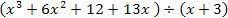: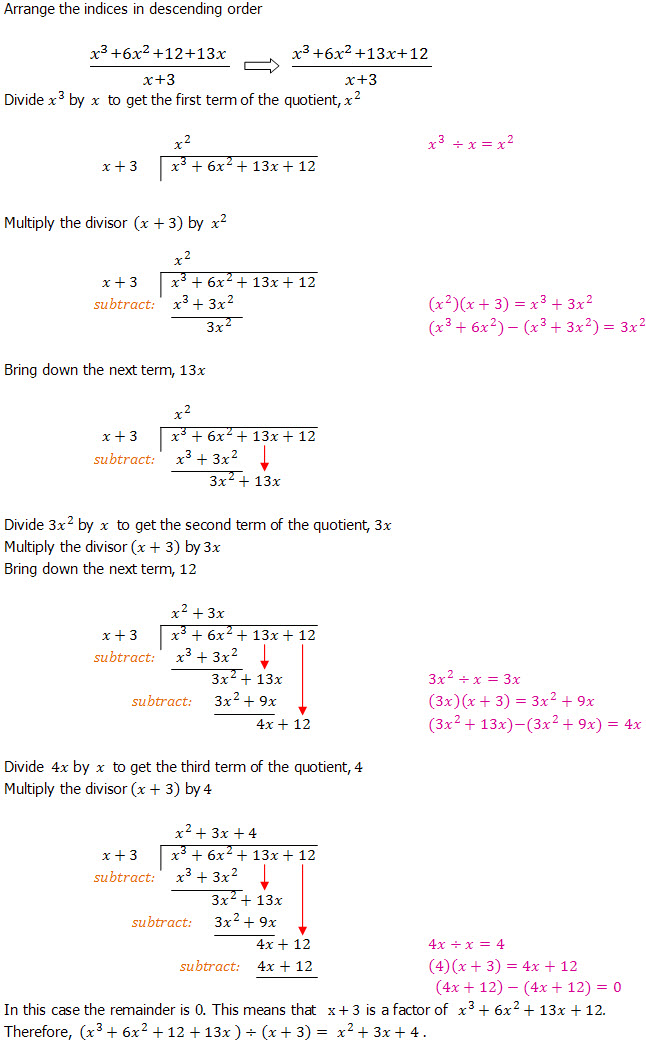Perform this long division: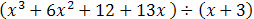:Perform this long division: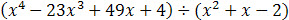: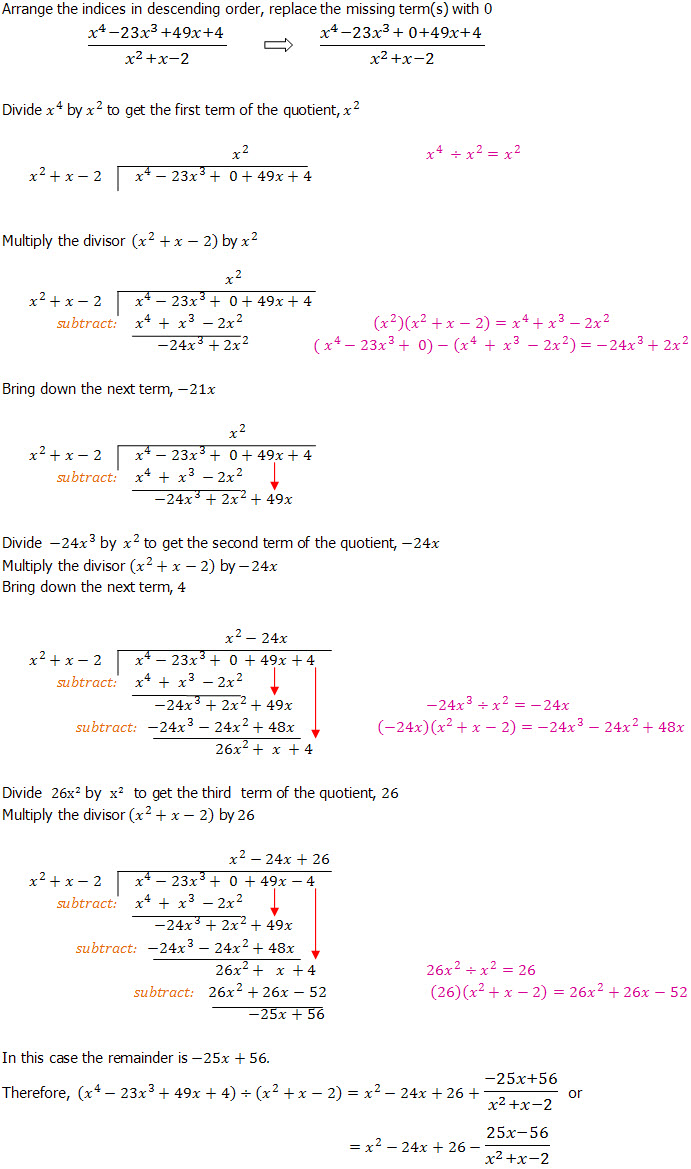Go to the next page to start practicing what you have learnt.